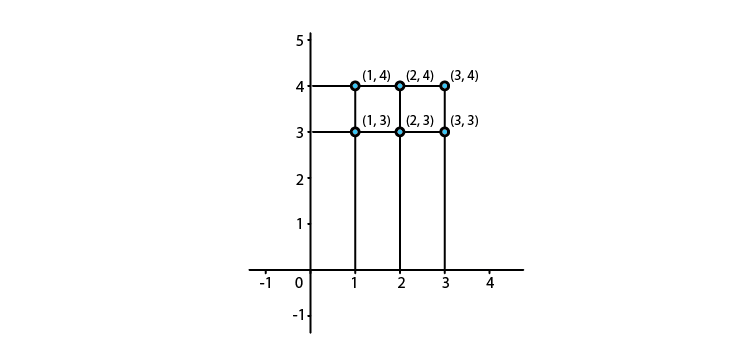Let A = {1, 2, 3} and B = {3, 4}. Find A x B and show it graphically

Asked by Aaryan | 1 year ago |  43

##### Solution :-

Given:

A = {1, 2, 3} and B = {3, 4}

A x B = {1, 2, 3} × {3, 4}

= {(1, 3), (1, 4), (2, 3), (2, 4), (3, 3), (3, 4)}

Steps to follow to represent A × B graphically,

Step 1: One horizontal and one vertical axis should be drawn

Step 2: Element of set A should be represented in horizontal axis and on vertical axis elements of set B should be represented

Step 3: Draw dotted lines perpendicular to horizontal and vertical axes through the elements of set A and B

Step 4: Point of intersection of these perpendicular represents A × BAnswered by Sakshi | 1 year ago

### Related Questions

#### Let R = {(a, b) : a, b, ϵ N and a < b}.Show that R is a binary relation on N

Let R = {(a, b) : a, b, ϵ N and a < b}.Show that R is a binary relation on N, which is neither reflexive nor symmetric. Show that R is transitive.

#### Let A = (1, 2, 3} and B = {4} How many relations can be defined from A to B.

Let A = (1, 2, 3} and B = {4} How many relations can be defined from A to B.

#### Let R = {(x, x2) : x is a prime number less than 10}.

Let R = {(x, x2) : x is a prime number less than 10}.

(i) Write R in roster form.

(ii) Find dom (R) and range (R).